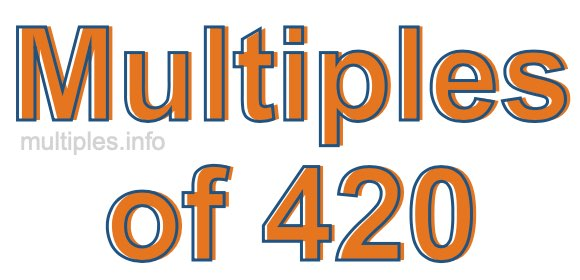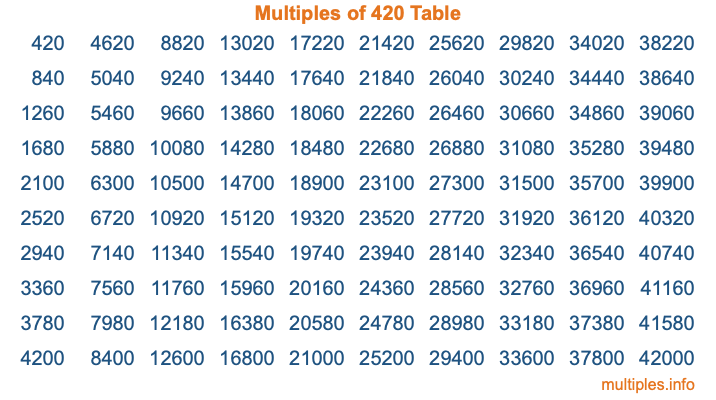Multiples of 420Welcome to the Multiples of 420 page. Here we will first teach you everything you will ever need to know about the multiples of 420, and then give you a study guide summary of everything we taught you to make sure you remember it all. Use this page to look up facts and learn information about the multiples of 420. This page will make you a multiples of four hundred twenty expert!

Definition of Multiples of 420
Multiples of 420 are all the numbers that when divided by 420 equal an integer. Each of the multiples of 420 are called a multiple. A multiple of 420 is created by multiplying 420 by an integer.

Therefore, to create a list of multiples of 420, you start with 1 multiplied by 420, then 2 multiplied by 420, then 3 multiplied by 420, and so on for as long as you want. Thus, the list of the first five multiples of 420 is 420, 840, 1260, 1680, and 2100. To see a larger list of multiples of 420, see the printable image of Multiples of 420 further down on this page. We also have a category where you can choose any nth multiple of 420.

Multiples of 420 Checker
The Multiples of 420 Checker below checks to see if any number of your choice is a multiple of 420. In other words, it checks to see if there is any number (integer) that when multiplied by 420 will equal your number. To do that, we divide your number by 420. If the the quotient is an integer, then your number is a multiple of 420.

Is  a multiple of 420?

Least Common Multiple of 420 and ...
A Least Common Multiple (LCM) is the lowest multiple that two or more numbers have in common. This is also called the smallest common multiple or lowest common multiple and is useful to know when you are adding our subtracting fractions. Enter one or more numbers below (420 is already entered) to find the LCM.

Check out our LCM Calculator if you need more details about the Least Common Multiple or if you need the LCM for different numbers for adding and subtraction fractions.

nth Multiple of 420
As we stated above, 420 is the first multiple of 420, 840 is the second multiple of 420, 1260 is the third multiple of 420, and so on. Enter a number below to find the nth multiple of 420.

th multiple of 420

Multiples of 420 vs Factors of 420
420 is a multiple of 420 and a factor of 420, but that is where the similarities end. All postive multiples of 420 are 420 or greater than 420. All positive factors of 420 are 420 or less than 420.

Below is the beginning list of multiples of 420 and the factors of 420 so you can compare:

Multiples of 420: 420, 840, 1260, 1680, 2100, etc.

Factors of 420: 1, 2, 3, 4, 5, 6, 7, 10, 12, 14, 15, 20, 21, 28, 30, 35, 42, 60, 70, 84, 105, 140, 210, 420

As you can see, the multiples of 420 are all the numbers that you can divide by 420 to get a whole number. The factors of 420, on the other hand, are all the whole numbers that you can multiply by another whole number to get 420.

It's also interesting to note that if a number (x) is a factor of 420, then 420 will also be a multiple of that number (x).

Multiples of 420 vs Divisors of 420
The divisors of 420 are all the integers that 420 can be divided by evenly. Below is a list of the divisors of 420.

Divisors of 420: 1, 2, 3, 4, 5, 6, 7, 10, 12, 14, 15, 20, 21, 28, 30, 35, 42, 60, 70, 84, 105, 140, 210, 420

The interesting thing to note here is that if you take any multiple of 420 and divide it by a divisor of 420, you will see that the quotient is an integer.

Multiples of 420 Table
Below is an image of the first 100 multiples of 420 in a table. The table is in chronological order, column by column. The first column has the first ten multiples of 420, the second column has the next ten multiples of 420, and so on.The Multiples of 420 Table is also referred to as the 420 Times Table or Times Table of 420. You are welcome to print out our table for your studies.

Negative Multiples of 420
Although not often discussed or needed in math, it is worth mentioning that you can make a list of negative multiples of 420 by multiplying 420 by -1, then by -2, then by -3, and so on, to get the following list of negative multiples of 420:

-420, -840, -1260, -1680, -2100, etc.

Multiples of 420 Summary
Below is a summary of important Multiples of 420 facts that we have discussed on this page. To retain the knowledge on this page, we recommend that you read through the summary and explain to yourself or a study partner why they hold true.

There are an infinite number of multiples of 420.

A multiple of 420 divided by 420 will equal a whole number.

420 divided by a factor of 420 equals a divisor of 420.

The nth multiple of 420 is n times 420.

The largest factor of 420 is equal to the first positive multiple of 420.

420 is a multiple of every factor of 420.

420 is a multiple of 420.

A multiple of 420 divided by a divisor of 420 equals an integer.

420 divided by a divisor of 420 equals a factor of 420.

Any integer times 420 will equal a multiple of 420.

Multiples of a Number
Here you can get the multiples of another number, all with the same attention to detail as we did for multiples of 420 on this page.

Multiples of
Multiples of 421
Did you find our page about multiples of four hundred twenty educational? Do you want more knowledge? Check out the multiples of the next number on our list!